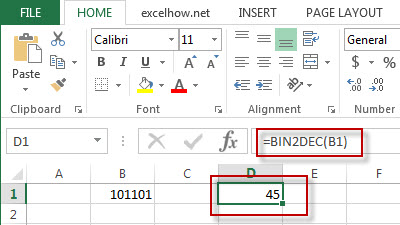## Excel Convert Function

This post will guide you how to use Excel CONVERT function with syntax and examples in Microsoft excel.

### Description

The Excel CONVERT function converts a number from one measurement system to another.

The CONVERT function is a build-in function in Microsoft Excel and it is categorized as an Engineering Function.

The CONVERT function is available in Excel 2016, Excel 2013, Excel 2010, Excel 2007, Excel 2003, Excel XP, Excel 2000, Excel 2011 for Mac.

### Syntax

The syntax of the CONVERT function is as below:

= CONVERT (number,from_unit,to_unit)

Where the CONVERT function argument is:
Number -This is a required argument. The value to be converted.
From_unit -This is a required argument. The units for number
To_unit -This is a required argument. The units for the result.

 Distance From_unit or to_unit Meter “m” Statute mile “mi” Nautical mile “Nmi” Inch “in” Foot “ft” Yard “yd” Angstrom “ang” Ell “ell” Light-year “ly” Parsec “parsec” or “pc” Pica (1/72 inch) “Picapt” or “Pica” Pica (1/6 inch) “pica” U.S survey mile (statute mile) “survey_mi”

The others Unit values can be referred to the official site https://support.office.com/en-us/article/CONVERT-function-d785bef1-808e-4aac-bdcd-666c810f9af2)

### Example

The below examples will show you how to use Excel CONVERT Function.

#1 =CONVERT(A1, “m”, “in”)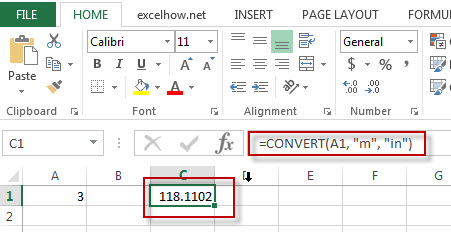Note: The above excel formulas will convert the value of B1 cell from “meter” unit to “Inch”.

## Excel Complex Function

This post will guide you how to use Excel COMPLEX function with syntax and examples in Microsoft excel.

### Description

The Excel COMPLEX function converts real and imaginary coefficients into a complex number.

The COMPLEX function is a build-in function in Microsoft Excel and it is categorized as an Engineering Function.

The COMPLEX function is available in Excel 2016, Excel 2013, Excel 2010, Excel 2007, Excel 2003, Excel XP, Excel 2000, Excel 2011 for Mac.

### Syntax

The syntax of the COMPLEX function is as below:

= COMPLEX (real_num,i_num,[suffix])

Where the COMPLEX function argument is:
Real_num -This is a required argument. The real coefficient of the complex number.
I_num -This is a required argument. The imaginary coefficient of the complex number.
Note:
If real_num value is not a numeric value, it will return a #VALUE! Error.
If i_num value is not a numeric value, it will return a #VALUE! Error.
If suffix is not either “i” nor “j”, it will return a #VALUE! Error.

### Example

The below examples will show you how to use Excel COMPLEX Function to convert coefficients into a complex number.

#1 =COMPLEX(A2,B2)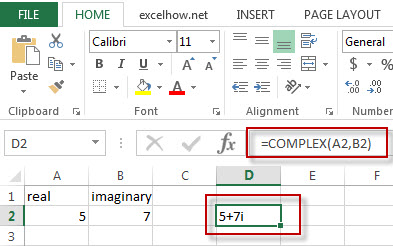## Excel Bin2Oct Function

This post will guide you how to use Excel BIN2OCT function with syntax and examples in Microsoft excel.

### Description

The Excel BIN2OCT function converts a binary number to octal.

The BIN2OCT function is a build-in function in Microsoft Excel and it is categorized as an Engineering Function.

The BIN2OCT function is available in Excel 2016, Excel 2013, Excel 2010, Excel 2007, Excel 2003, Excel XP, Excel 2000, Excel 2011 for Mac.

### Syntax

The syntax of the BIN2OCT function is as below:

= BIN2OCT (binary_number)

Where the BIN2OCT function argument is:
Binary_number -This is a required argument. The binary number that you want to convert to octal.

### Example

The below examples will show you how to use Excel BIN2OCT Function to convert a binary number to a octal number.

#1 = BIN2OCT (B1)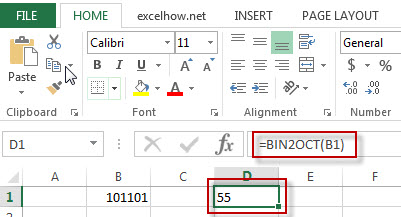## Excel Bin2Hex Function

This post will guide you how to use Excel BIN2HEX function with syntax and examples in Microsoft excel.

### Description

The Excel BIN2HEX function converts a binary number to hexadecimal.

The BIN2HEX function is a build-in function in Microsoft Excel and it is categorized as an Engineering Function.

The BIN2HEX function is available in Excel 2016, Excel 2013, Excel 2010, Excel 2007, Excel 2003, Excel XP, Excel 2000, Excel 2011 for Mac.

### Syntax

The syntax of the BIN2HEX function is as below:

= BIN2HEX (binary_number)

Where the BIN2HEX function argument is:
Binary_number -This is a required argument. The binary number that you want to convert to hexadecimal.

### Example

The below examples will show you how to use Excel BIN2HEX Function to convert a binary number to a hexadecimal number.

#1 = BIN2HEX (B1)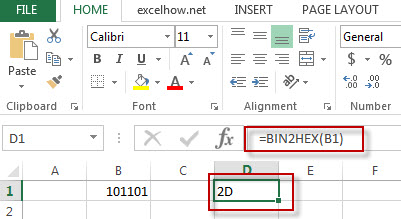## Excel Bin2Dec Function

This post will guide you how to use Excel BIN2DEC function with syntax and examples in Microsoft excel.

### Description

The Excel BIN2DEC function converts a binary number to decimal.

The BIN2DEC function is a build-in function in Microsoft Excel and it is categorized as an Engineering Function.

The BIN2DEC function is available in Excel 2016, Excel 2013, Excel 2010, Excel 2007, Excel 2003, Excel XP, Excel 2000, Excel 2011 for Mac.

### Syntax

The syntax of the BIN2DEC function is as below:

= BIN2DEC (binary_number)

Where the BIN2DEC function argument is:
Binary_number -This is a required argument. The binary number that you want to convert to decimal.

### Example

The below examples will show you how to use Excel BIN2DEC Function to convert a binary number to a decimal number.

#1 = BIN2DEC (B1)